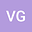A new extension of the (H.2) supercongruence of Van Hamme for primes $p\equiv 3\pmod{4}$
•Using Andrews’ multi-series generaliazation of Watson’s $_8\phi_7$ transformation, we give a new extension of the (H.2) supercongruence of Van Hamme for primes $p\equiv 3\pmod{4}$, as well as its $q$-analogue. Meanwhile, applying the method of ‘creative microscoping’, recently introduced by the author and Zudilin, we establish some further $q$-supercongruences modulo $\Phi_n(q)^3$, where $\Phi_n(q)$ denotes the $n$-th cyclotomic polynomial in $q$.